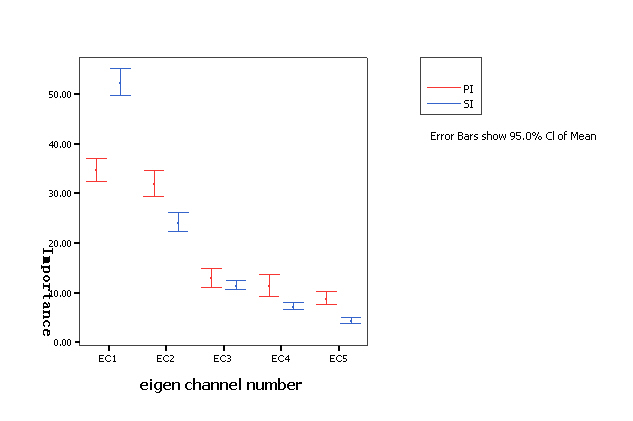# Perceptual importance of KLT eigenchannels

The Karhunen-Leve Transform (KLT) can be used to reduce the interchannel redundancy of multichannel audio signals due to its mathematical properties as KLT can transform a set of correlated signals into a set of uncorrelated signals (Yang, 2003). In addition to signal decorrelation, KLT has another very valuable property: the KL-transformed signals are ordered hierarchically according to the amount of signal variance they explain. However, it cannot be directly deduced that the mathematical character of KLT will lead to the same effect on people's perception of the quality of multichannel audio. The perceptual importance of Karhunen- Leve transformed multichannel audio signals was systematically studied using listening tests. Three eigenvalue extraction methods were employed as follows: a covariance matrix based eigenvalue extraction (Cov-KLT), a correlation matrix based eigenvalue extraction (Corr-KLT) and a perceptually optimised eigenvalue extraction method (PO-KLT). Conclusions can be summarised as follows:

1. Basic audio quality decreased as the number of removed eigenchannels increased.

2. KLT eigenchannel removal caused less degradation in audio quality for multichannel audio with high interchannel correlation than for those items with low interchannel correlation.

3. Three eigenvalue extraction methods resulted in similar performance of BAQ for FF and FF+C recordings. But for FB and FB+C recordings, the KLT processing based on covariance matrix (Cov-KLT) and perceptually optimised covariance matrix (PO-KLT) caused smaller audio quality degradation than that caused by the KLT processing based on correlation matrix (Corr-KLT).

4. In general, for Corr-KLT and Cov-KLT, the orders of perceptual importance (PI) and statistical importance (SI) of eigenchannels are the same, whereas for the PO-KLT, the perceptual importance of the 2 nd eigenchannel is higher than the 1 st eigenchannel (see Figure 1). However, it is difficult to generalise these observations as the results strongly depend on programme material.Figure 1: Comparison between Perceptual Importance (PI) and Statistical Importance (SI) of eigenchannels

This work is reported in detail in [P2].